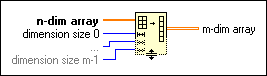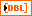# Reshape Array Function

## LabVIEW 2018 Help

Edition Date: March 2018
Part Number: 371361R-01
View Product InfoLabVIEW 2016 HelpLabVIEW 2017 HelpLabVIEW 2018 HelpLabVIEW 2019 HelpLabVIEW 2020 Help

Owning Palette: Array Functions

Requires: Base Development System

Changes the dimensions of an array according to the values of dimension size 0..m-1.

This function reads the array data in memory from left to right, row by row and populates the reshaped array the same way. For example, if you pass a 2D array of 8 elements, 2 columns of {0,1,2, 3}, to this function with two dimensions defined with sizes of 2 and 4, respectively, the function returns an array containing {{0,0,1,1}, {2,2, 3, 3}}. The connector pane displays the default data types for this polymorphic function.n-dim array can be an n-dimensional array of any type.dimension size 0..m-1 specifies the dimensions of m-dim array and must be a numeric. The function creates an empty array if any dimension size is 0. You must have m dimension size terminals for m-dimensions.m-dim array If the product of the dimension sizes is greater than the number of elements in the input array, the function pads the new array with the default of the data type of n-dim array. If the product of the dimension sizes is less than the number of elements in the input array, the function truncates the array.

## Reshape Array Details

This function adjusts the array data in memory according to the reshaped array dimensions. This function pads or truncates the data if necessary. For example, if you pass a 1D array with 9 elements {0, 1, 2, 3, 4, 5, 6, 7, 8} to this function with two dimensions defined with sizes of 2 and 3, respectively, the function returns a 2D array containing {{0, 1, 2}, {3, 4, 5}}. The function truncates the last three input elements because the output array has room for only six values.

Resize this function to increase the number of dimension size parameters. m-dim array has one dimension for each dimension size input. For example, you can use this function to change a 1D array into a 2D array or vice versa. You also can use it to increase and decrease the size of a 1D array.